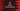# C# program to find the largest of three numbers### C# program to find the largest of three numbers:

In this C# program, we will learn how to find the largest of three numbers. The program will ask the user to enter these numbers one by one and then it will find the largest of three numbers and print it out.

We will learn two different ways to solve this problem. The first one will use one basic way to solve it by comparing the numbers and the other one will use one system function to find out the max of two.

### Method 1: By using simple comparison:

Let’s solve it by using a simple comparison. Below is the algorithm for that:

• Get the numbers.
• Check the largest of first two.
• If first > second, compare first with third and find the largest.
• Else, compare second with third and find the largest.

Below is the complete program:

``````using System;
namespace dotnet_sample
{
class Program
{
static void Main(string[] args)
{
//1
int first, second, third;

//2
Console.WriteLine("Enter the first number : ");

Console.WriteLine("Enter the second number : ");

Console.WriteLine("Enter the third number : ");

//3
if (first > second)
{
//4
if (first > third)
{
Console.WriteLine("largest number : " + first);
}
else
{
Console.WriteLine("largest number : " + third);
}
}
else
{
if (second > third)
{
Console.WriteLine("largest number : " + second);
}
else
{
Console.WriteLine("largest number : " + third);
}
}
}
}
}``````

### Explanation:

The commented numbers in the above program denote the step numbers below :

1. Create three integer variables to store the user inputs.
2. Ask the user to enter the numbers. Read and store these in the variables.
3. Check if first > third. If yes, then compare first with third and print out the largest number. Else, check second with third and print the largest.

### Sample Output:

``````Enter the first number :
10
Enter the second number :
5
Enter the third number :
4
largest number : 10``````

### Method 2: By using Math.max():

The above process takes a lot of lines and checks to find out the max value. Instead, we can also use Math.max() function that is already defined. Math.max() takes two parameters and returns the max. So, we can use it two times to find out the maximum of three.

``````using System;
namespace dotnet_sample
{
class Program
{
static void Main(string[] args)
{
int first, second, third;

Console.WriteLine("Enter the first number : ");

Console.WriteLine("Enter the second number : ");

Console.WriteLine("Enter the third number : ");

int maxValue = Math.Max(Math.Max(first,second),third);

Console.WriteLine("Largest value : "+maxValue);
}
}
}``````

As you can see here, we are finding the maximum of three numbers in just one line :)

If you run this program, it will give the same output.

C# find largest of three numbers